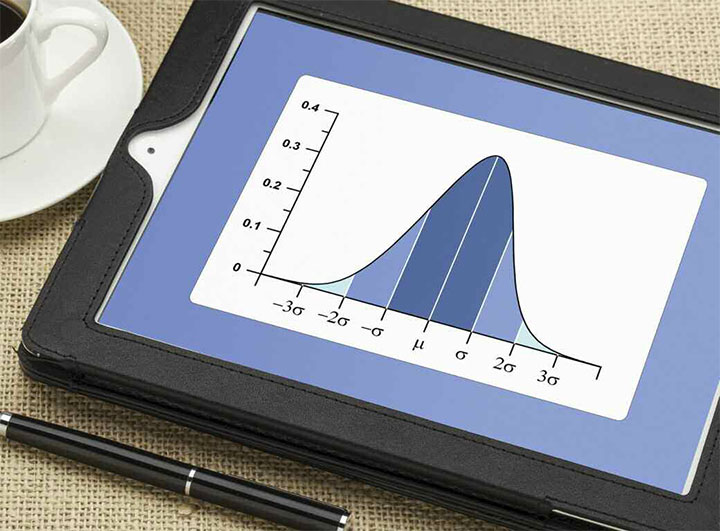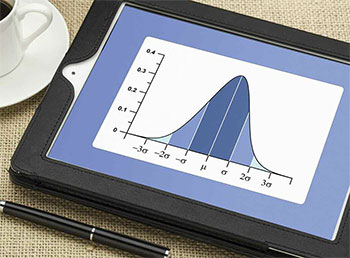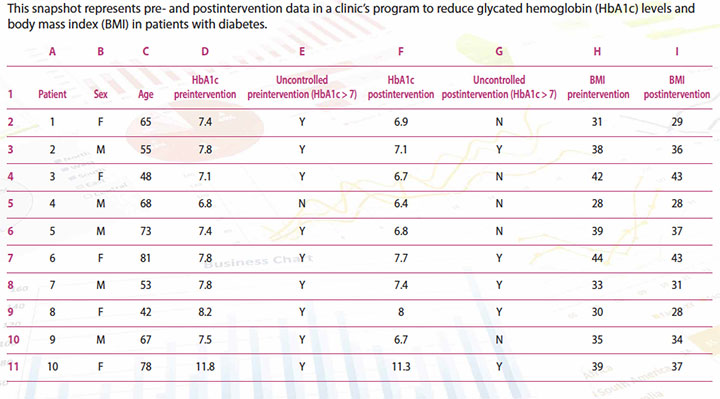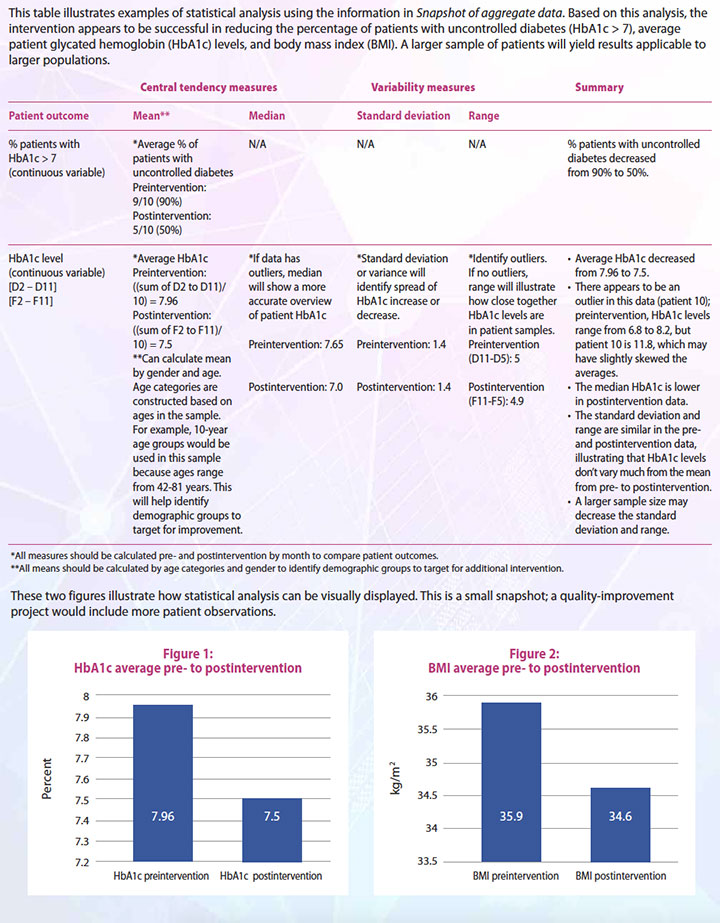# Research 101: Descriptive statistics

Author(s): Brian Conner, PhD, RN, CNE and Emily Johnson, PhD## Use these tools to analyze data vital to practice-improvement projects.

By Brian Conner, PhD, RN, CNE and Emily Johnson, PhD

Takeaways:

• Nurses at every level should be able to understand and apply basic statistical analyses related to performance improvement projects.
• Measures of central tendency (such as mean) and variability (such as standard deviation) are fairly common and easy to use.

How many times have you said (or heard), “Statistics are too complicated”? A significant percentage of graduate students and nurses in clinical practice report feeling anxious when working with statistics. And although some statistical analysis is pretty complicated, you don’t need a doctoral degree to understand and use descriptive statistics.

## What are descriptive statistics?Descriptive statistics are just what they sound like—analyses that summarize, describe, and allow for the presentation of data in ways that make them easier to understand. They help us understand and describe the aspects of a specific set of data by providing brief observations and summaries about the sample, which can help identify patterns. The summaries typically involve quantitative data and visuals such as graphs and charts.

Sometimes, descriptive statistics are the only analyses completed in a research or evidence-based practice study; however, they don’t typically help us reach conclusions about hypotheses. Instead, they’re used as preliminary data, which can provide the foundation for future research by defining initial problems or identifying essential analyses in more complex investigations.

## Common descriptive statistics

The most common types of descriptive statistics are the measures of central tendency (mean, median, and mode) that are used in most levels of math, research, evidence-based practice, and quality improvement. These measures describe the central portion of frequency distribution for a data set.

The most familiar of these is the mean, or average, which most people use and understand. It’s calculated by adding the sum of values in the data and dividing by the total number of observations.

The median is a number found at the exact middle of a set of data. If there are two numbers at the middle of the data set (which occurs when there is an even number of data points), these two numbers are averaged to identify the median. It’s typically used to describe a data set that has extreme outliers (very low or very high numbers, distant from the majority of data points), in which case the mean will not accurately represent the data. (See What to do with outliers.) To calculate a mean or median, data must be quantitative/continuous (have an infinite number of possibilities).

## What to do with outliers

 When analyzing descriptive statistics, watch for outliers. These data points are distant from the majority of observations and may be the result of measurement error, coding error, or extreme variability in an observation. In addition to visually perusing data for outliers, you can identify them using graphical display and complex modeling. Depending on the number of outliers, they’re either statistically transformed (using a complex statistical formula to balance all variable values) or excluded from the data set.

The mode represents the most frequently occurring number or item in a data set. Some data sets have more than one mode, making them bimodal (two modes) or multimodal (more than two modes). The mode can be calculated with data that are quantitative/continuous or qualitative/categorical (have a finite number of categories or groups, such as sex, race, or education level). The mode is the only measure of central tendency that can be analyzed with qualitative/categorical data.

## Less common descriptive statistics

Measures of variability or dispersion are less common descriptive statistics, but they’re still important because they describe the spread of values across a data set. Although the central tendency of data is vital, the range of values (the difference between the maximum and minimum values in the data) also may be important to note. The range not only sets boundaries for your data set and indicates the spread, but it also can identify errors in the data. For example, if you have a data set with a diastolic blood pressure range of 230 (highest diastolic value) to 25 (lowest diastolic value) = 205 (range), an error probably exists in your data because the values of 230 and 25 aren’t valid blood pressure measures in most studies. Other measures of variability include standard deviation, variance, and quartiles. (See Other variability measures.)

## Other variability measures

 Standard deviation, variance, and quartile can be used in addition to range to measure variability of data. Standard deviation is the average distance of each data point from the mean of the data set. It’s calculated by taking the square root of the sum of all numbers minus the mean (squared) and dividing by one less than the number of values. For example, in a data set of five systolic blood pressures of 125, 128, 142, 145, and 150, the mean would be 138, based on this calculation: (125+128+142+145+150)/5. The standard deviation would be 10.9, based on this calculation: √(((125-138)2 + (128-138)2 + (142-138)2 + (145-138)2 + (150-138)2)/(5-1)), indicating that there’s not a large dispersion in this set of systolic measures. (Don’t worry about the complexity of the formula; you can enter the data points in a free standard deviation tool that does the calculation for you. The formula is here to illustrate the point.) The variance also describes the variation of data points from the mean, but it’s affected by outliers. If the standard deviation and variance are large, the spread of data points in the data set also is large; however, if the standard deviation and variance are small, most data points are close to the mean. Whether standard deviation and variance are determined to be small or large depends on the range of data. For example, in data with a range of 5, a standard deviation of 4 would be large; however, in data with a range of 10,000, a standard deviation of 4 would be small. A quartile (q) consists of three points, q1 (lower), q2 (median), and q3 (upper), that divide a list of numbers into four equal categories. When using quartiles, you can identify the interquartile range (q3-q1), which describes the middle part of the data set. Range, standard deviation, variance, and quartiles are all used with quantitative/continuous data, but they can’t be used to analyze qualitative/categorical data.

## Practical application of descriptive statistics

To put all of this information into perspective, here’s an example of how these measures can be used in a clinical setting.

A rural primary care clinic has a high percentage of patients with diabetes whose glycated hemo­globin (HbA1c) levels are greater than 7% (uncontrolled HbA1c) and body mass index (BMI) is over 30. The clinic implements a 9-month quality-improvement initiative to lower these numbers. The initiative includes a wellness education program focused on exercise, healthy eating, and understanding the importance of regular blood glucose monitoring. Before implementing the program, the clinic collects 3 months of aggregate data (3, 6, and 9 months before the intervention) for all patients with diabetes in the clinic, including HbA1c levels, BMIs, and patients with uncontrolled HbA1c. Gender and age also are collected. The clinic then collects the same data 3, 6, and 9 months after implementation of the program. (See Snapshot of aggregate data.) Because of the different types of data collected, different measures of central tendency and variability can help describe outcomes. (See Statistical analysis examples.)

## Snapshot of aggregate data## Statistical analysis examples## Implications for practice

Nurses are increasingly asked to participate and lead evidence-based practice and quality-improvement projects. Many healthcare organizations, including those aspiring to or holding Magnet® recognition from the American Nurses Credentialing Center, require that nurses take part in these activities to achieve higher levels of professional development within clinical ladder programs. Nurses can and should learn how to use descriptive statistics to analyze and depict vital data related to practice-improvement projects.

Brian Conner is adjunct faculty at the School of Nursing and Health Sciences for Simmons College in Boston, Massachusetts. Emily Johnson is an assistant professor at the Medical University of South Carolina College of Nursing in Charleston.

## Selected references

American Nurses Credentialing Center (ANCC). Magnet Recognition Program® Overview. 2016.

Heavey E. Statistics for Nursing: A Practical Approach. 2nd ed. Burlington, MA: Jones and Bartlett Learning; 2015.

Thabane L, Akhtar-Danesh N. Guidelines for reporting descriptive statistics in health research. Nurse Res. 2008;15(2):72-81.

Zhang Y, Shang L, Wang R, et al. Attitudes toward statistics in medical postgraduates: Measuring, evaluating and monitoring. BMC Med Educ. 2012;12:117.

ant11-Research 101-1017a

#### 1 COMMENT

1. This is a great data set and I would like to see if I can have copy right to use it in my « statistical thinking for Nursing » course here at Castleton Unvesity to demonstrate Bootsrapjng technique as Inferential statistics. Please let me know. Thank You
Dr. Rajia